Reporting Forum
1.1K+ views

Hello Supports,

I want to try and install Nuget packages Telerik.Reporting.Services.AspNetCore for my Asp.net Core project but it isn't found.

when I go to the option "Manage Nuget packages", these are no available the packages to download (see the attached file).
Is it missing from the private nuget feed ?

Thank You.Marc
Top achievementsRank 2Iron
5 views

We have a .NET Web Application hosted in Azure. We have recently had reports of issues opening telerik reports with the error: An exception was thrown by a TaskScheduler. Exception of type "System.OutOfMemory" was thrown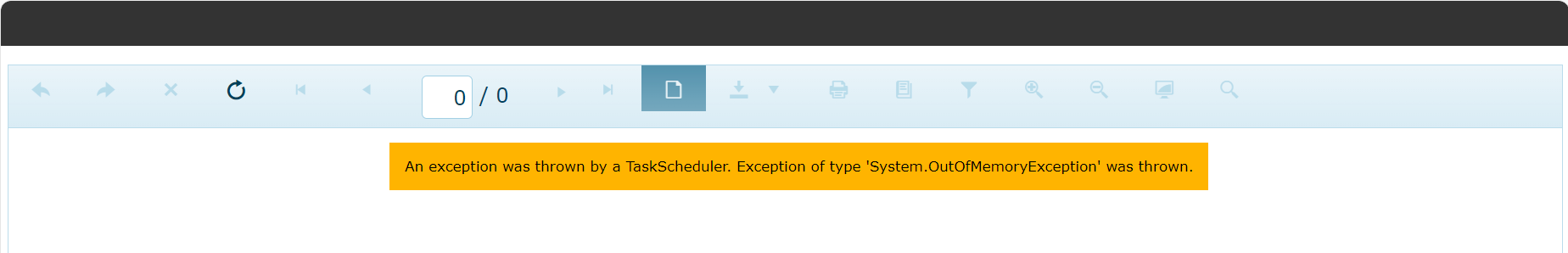Todor
Telerik team3 views

I have a report that I am trying to get the number of rows displayed.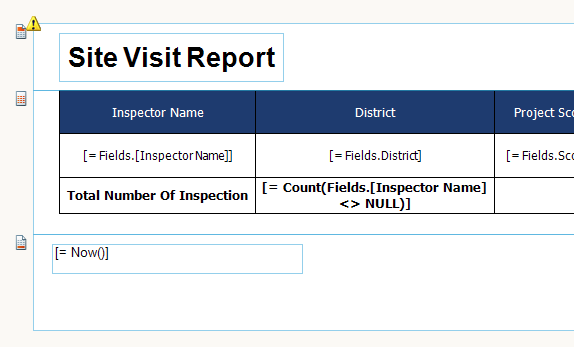Which displays: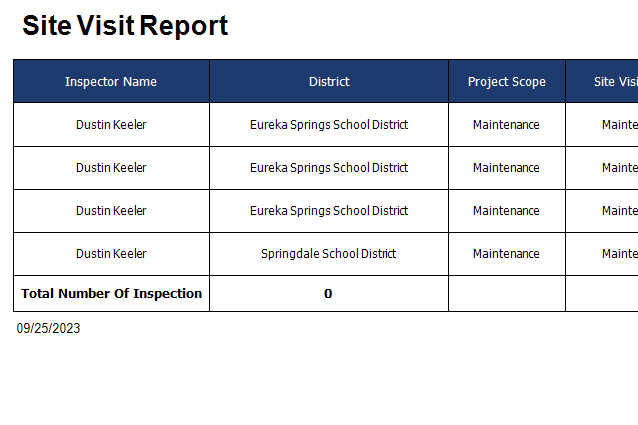I have also tried = Count(Fields.[Inspector Name])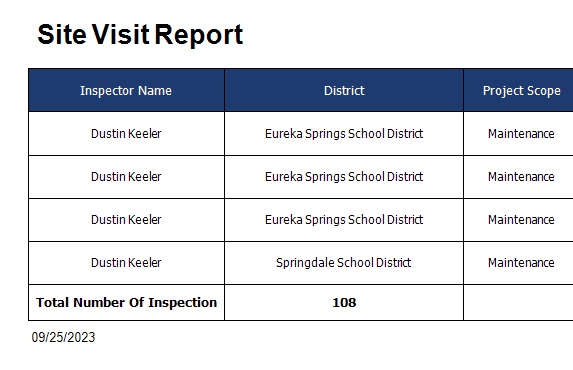It should only show the 4 for each visible row. What have I done wrong?Randy
Top achievementsRank 1
5 views

Dear Team,

I using telerik report Angular report viewer version 15.0.21.326 , I clicked on export button but no response "export to pdf".May I know how can i fix this issue ?

Thank youLEON
Top achievementsRank 1
10 views

Dear Team,

We have to evaluate an old project which uses Telerik Reporting Version Q3.2020. The task is to migrate and update older asp.net and Windows forms reports to newer report version.

Thank you,

AnupLance | Manager Technical Support
Telerik team6 views

I have a complicated expression that I'd like to set a lower bound to.

The value should never dip below 34.

The only working solution I've found is:

Let f(x) be the expression used to calculate the value.

To include a lower bound I've been doing "=IF(f(x) < 34, 34, f(x))"

This works well when f(x) is simple but this explodes in length as the length of f(x) increases.

If I wanted to also include an upper bound of 50 I would need to write the expression as

"=IF(f(x) < 34, 34 IF(f(x) > 50, 50, f(x)))"

I've tried the Min function but it just remains blank.

"=Min(Array(5,7))" does not return 5

For context here is the expression I created to calculate the proper zoom level for a static google map.

Please note that these formulas are generated by another program I created to help me manage the complex calculations I need to do. There is no way I would ever try to manage these manually. Currently, there are 30 pins being managed and my boss is thinking about increasing this to 90.

Floor(IF(LOG(((((Parameters.PixelHeight)/2-(Parameters.Buffer))*Parameters.Pi/64))/ABS((LOG((1+(SIN((Max(X_COORD))*(Parameters.Pi)/180)))/(1-(SIN((Max(X_COORD))*(Parameters.Pi)/180))))/LOG(EXP(1)))-(LOG((1+(SIN(((Max(X_COORD)+Min(X_COORD))/2)*(Parameters.Pi)/180)))/(1-(SIN(((Max(X_COORD)+Min(X_COORD))/2)*(Parameters.Pi)/180))))/LOG(EXP(1)))))/LOG(2) < LOG(((((Parameters.PixelWidth)/2-(Parameters.Buffer))*360/256))/(Max(Y_COORD)-((Max(Y_COORD)+Min(Y_COORD))/2)))/LOG(2), LOG(((((Parameters.PixelHeight)/2-(Parameters.Buffer))*Parameters.Pi/64))/ABS((LOG((1+(SIN((Max(X_COORD))*(Parameters.Pi)/180)))/(1-(SIN((Max(X_COORD))*(Parameters.Pi)/180))))/LOG(EXP(1)))-(LOG((1+(SIN(((Max(X_COORD)+Min(X_COORD))/2)*(Parameters.Pi)/180)))/(1-(SIN(((Max(X_COORD)+Min(X_COORD))/2)*(Parameters.Pi)/180))))/LOG(EXP(1)))))/LOG(2), LOG(((((Parameters.PixelWidth)/2-(Parameters.Buffer))*360/256))/(Max(Y_COORD)-((Max(Y_COORD)+Min(Y_COORD))/2)))/LOG(2)))

Which has to be reused when I need to determine the position of a pin in my report.

200+256*POW(2,(Floor(IF(LOG(((((Parameters.PixelHeight)/2-(Parameters.Buffer))*Parameters.Pi/64))/ABS((LOG((1+(SIN((Max(X_COORD))*(Parameters.Pi)/180)))/(1-(SIN((Max(X_COORD))*(Parameters.Pi)/180))))/LOG(EXP(1)))-(LOG((1+(SIN(((Max(X_COORD)+Min(X_COORD))/2)*(Parameters.Pi)/180)))/(1-(SIN(((Max(X_COORD)+Min(X_COORD))/2)*(Parameters.Pi)/180))))/LOG(EXP(1)))))/LOG(2) < LOG(((((Parameters.PixelWidth)/2-(Parameters.Buffer))*360/256))/(Max(Y_COORD)-((Max(Y_COORD)+Min(Y_COORD))/2)))/LOG(2), LOG(((((Parameters.PixelHeight)/2-(Parameters.Buffer))*Parameters.Pi/64))/ABS((LOG((1+(SIN((Max(X_COORD))*(Parameters.Pi)/180)))/(1-(SIN((Max(X_COORD))*(Parameters.Pi)/180))))/LOG(EXP(1)))-(LOG((1+(SIN(((Max(X_COORD)+Min(X_COORD))/2)*(Parameters.Pi)/180)))/(1-(SIN(((Max(X_COORD)+Min(X_COORD))/2)*(Parameters.Pi)/180))))/LOG(EXP(1)))))/LOG(2), LOG(((((Parameters.PixelWidth)/2-(Parameters.Buffer))*360/256))/(Max(Y_COORD)-((Max(Y_COORD)+Min(Y_COORD))/2)))/LOG(2)))))*(((Item(1,AllValues(Y_COORD)))+180)/360) - 256*POW(2,(Floor(IF(LOG(((((Parameters.PixelHeight)/2-(Parameters.Buffer))*Parameters.Pi/64))/ABS((LOG((1+(SIN((Max(X_COORD))*(Parameters.Pi)/180)))/(1-(SIN((Max(X_COORD))*(Parameters.Pi)/180))))/LOG(EXP(1)))-(LOG((1+(SIN(((Max(X_COORD)+Min(X_COORD))/2)*(Parameters.Pi)/180)))/(1-(SIN(((Max(X_COORD)+Min(X_COORD))/2)*(Parameters.Pi)/180))))/LOG(EXP(1)))))/LOG(2) < LOG(((((Parameters.PixelWidth)/2-(Parameters.Buffer))*360/256))/(Max(Y_COORD)-((Max(Y_COORD)+Min(Y_COORD))/2)))/LOG(2), LOG(((((Parameters.PixelHeight)/2-(Parameters.Buffer))*Parameters.Pi/64))/ABS((LOG((1+(SIN((Max(X_COORD))*(Parameters.Pi)/180)))/(1-(SIN((Max(X_COORD))*(Parameters.Pi)/180))))/LOG(EXP(1)))-(LOG((1+(SIN(((Max(X_COORD)+Min(X_COORD))/2)*(Parameters.Pi)/180)))/(1-(SIN(((Max(X_COORD)+Min(X_COORD))/2)*(Parameters.Pi)/180))))/LOG(EXP(1)))))/LOG(2), LOG(((((Parameters.PixelWidth)/2-(Parameters.Buffer))*360/256))/(Max(Y_COORD)-((Max(Y_COORD)+Min(Y_COORD))/2)))/LOG(2)))))*((((Max(Y_COORD)+Min(Y_COORD))/2)+180)/360)Pascal
Top achievementsRank 1IronIron
6 views

I wasted hours trying to figure out what was wrong with my equations until I realized that Telerik moves panels around based on the visibility of other panels.

How can I stop this from happening? I want the pixel position of a panel to be static and not be influenced by other panels.

Instead of posting my report which has a mile long formula for determining the pixel position of several panels, I have found a very simple report to show this issue.

Drag and drop four panels next to each other. Set the background of each panel to a different colour.

Set the visibility of one of the middle panels to false and now preview. All panels to the right of the panel in question have now moved left.

Setting Panel2 to not be visible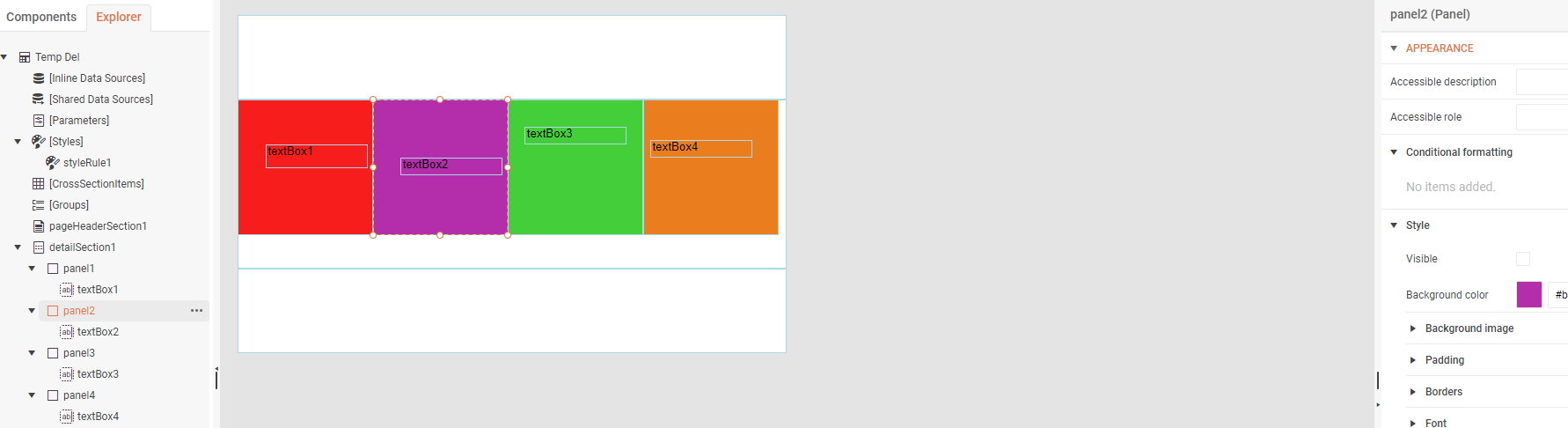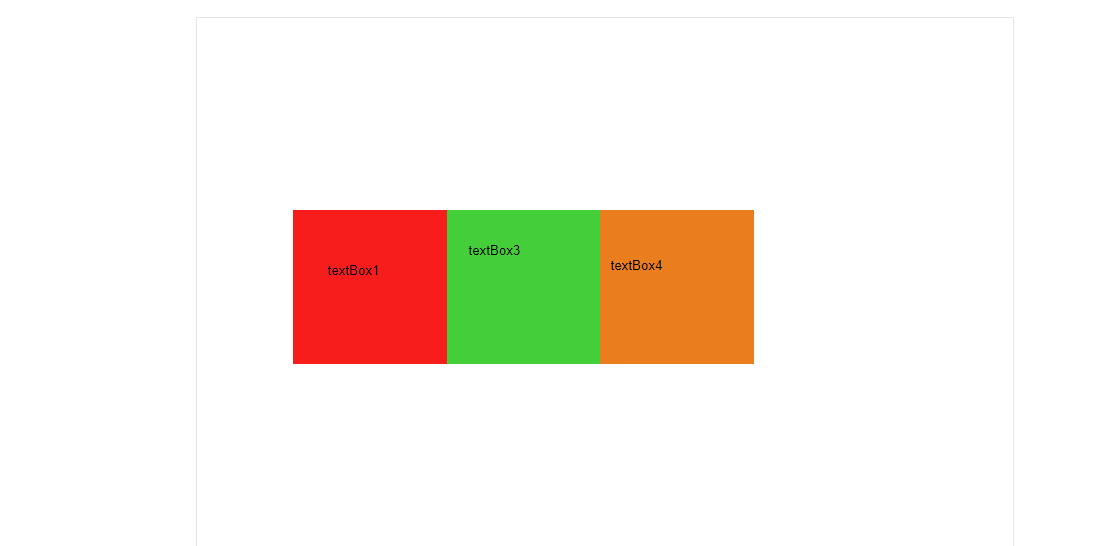Leaving Panel2 as visible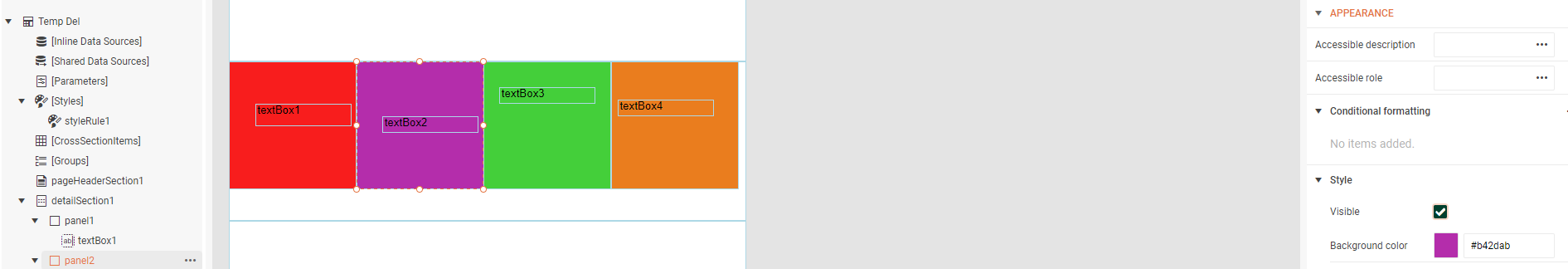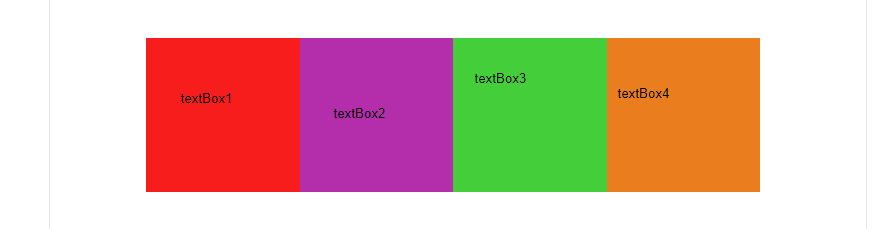Pascal
Top achievementsRank 1IronIron
13 views

Using Telerik.ReportViewer.BlazorNative 17.1.23.718

You cannot send an email with a blank CC!  Validation says that the email format is invalid.  I've looked through the docs and see no way of removing the CC field or any other way to make this work.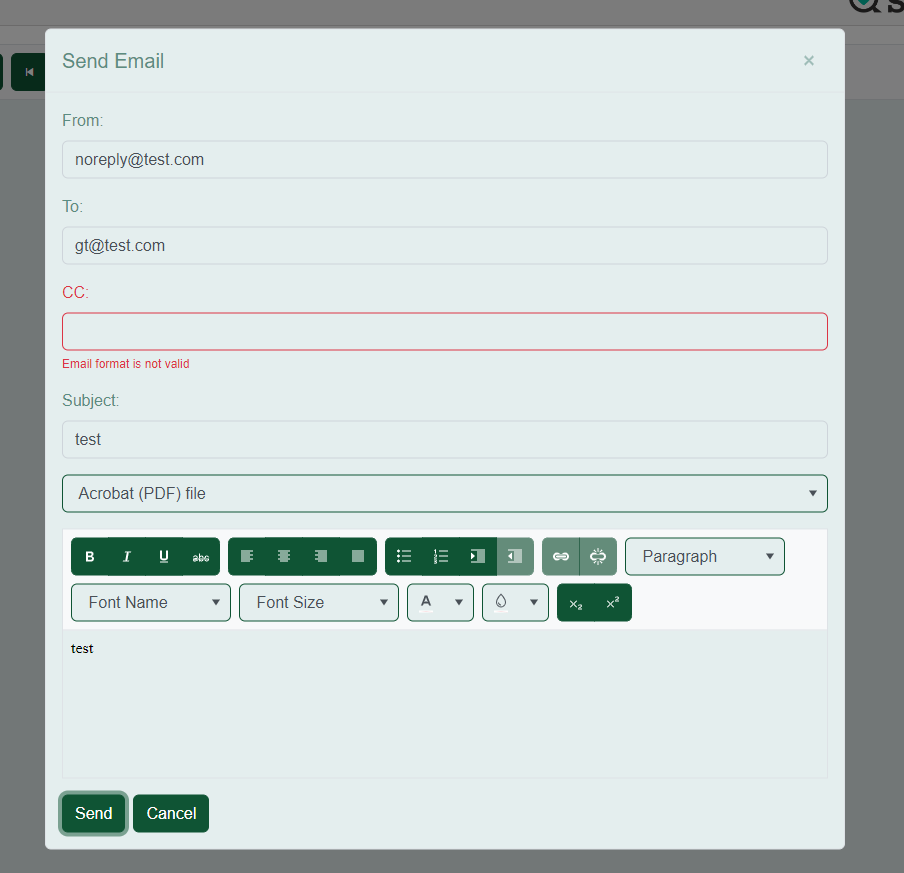Dimitar
Telerik team8 views

We are try to create reportservice project in c#.net core. but we are not able to get packages from nueget and even we try to add manually from example folder but its not  added. why i dont know please support

Telerik.Reporting.Services.AspNetCore.dll

Telerik.Reporting.OpenXmlRendering.2.7.2.dllArjun
Top achievementsRank 1
updated question on 19 Sep 2023
13 views

I have a comma delimited string parameter that is parsed into a c# datasource method when a Telerik report gets rendered. When I pass the string as it is into the datasource, using Parameters.personId.Value it fails and I get an error. When I use the Join(",", Parameters.personId.Value) function, it only works if the string has a single value and it's not comma delimited. The moment I append another value to the string, it sends the parameter as an empty string to the datasource.

This is how my data looks like:

Telerik UI parameter: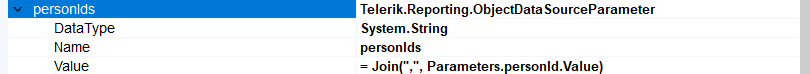C# DataSource definition: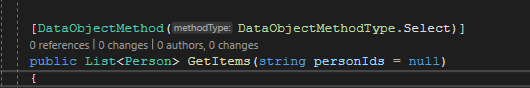Sample Parameter Value:

PersonIds: "965985,663958"

Thanks.Todor
Telerik teamSelected tags
Tags
+? more
Top users last monthBernd
Top achievementsRank 5BronzeBronzeIronkva
Top achievementsRank 2IronIronIronJay
Top achievementsRank 2IronIronVeteranMark
Top achievementsRank 3IronIronIronRuchika
Top achievementsRank 1IronWant to show your ninja superpower to fellow developers?